#### Archive

Category Archives for "Dip Singh"

## Introduction

Following up on the last post, we will explore IS-IS Area Proxy in this post. The main goal of the IS-IS Area proxy is to provide abstraction by hiding the topology. Looking at our toy topology, we see that we have fabrics connected, and the whole network is a single flat level-2 flooding domain. The edge nodes are connected at the ends, transiting multiple fabrics, and view all the nodes in the topology.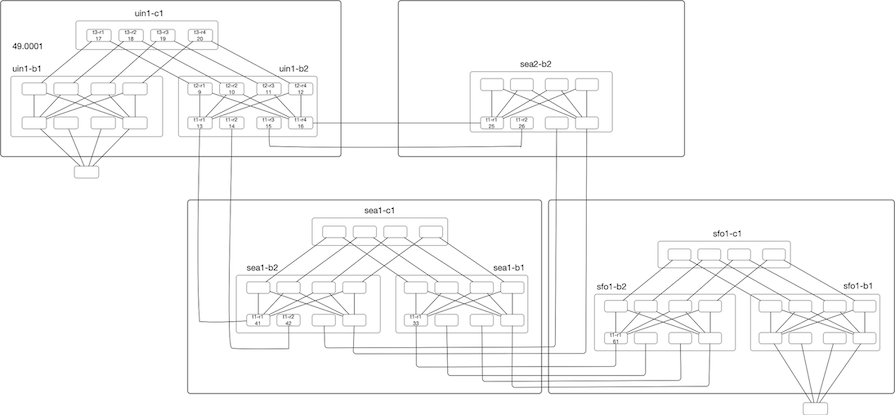Now assume that we are using a router with a radix of 32x100G and want to deploy three-level Fat-Tree(32,3). For a single fabric, we will have 1280 nodes, 512 leaf Nodes, providing a bandwidth of 819T. If we deploy ten instances of this fabric, we are looking at a topology size greater than >12k Nodes. This is a lot for any IGP to handle. This inflation of Nodes (and links) is coming from deploying this sort of dense topology to provide more bandwidth and directly impacts IGP scaling in terms of Flooding, LSDB size, SPF runtimes, and frequency of SPF run.

Referring back to our toy topology, if we look from the edge node’s perspective, they use these fabrics as transit, and if we can

## Introduction

In recent years, a lot of work has been done to scale IGPs for dense topologies, making IGPs again an interesting area. In this blog post, we will look at IS-IS Flooding and how we can measure the flooding rate, and in the future post explore Dynamic Flooding and Area Proxy.

### Topology Setup

For our experiment, we will use a stripped-down topology connecting Four locations. The devices are emulated using Arista cEOS, and all devices are part of a single level2 flooding domain. Topology creation was done with the help of netsim-tools and containerlabs. So my regards go to everyone involved with the tool, as it took care of the monotonous work like IP-Addressing, wiring, and base configs.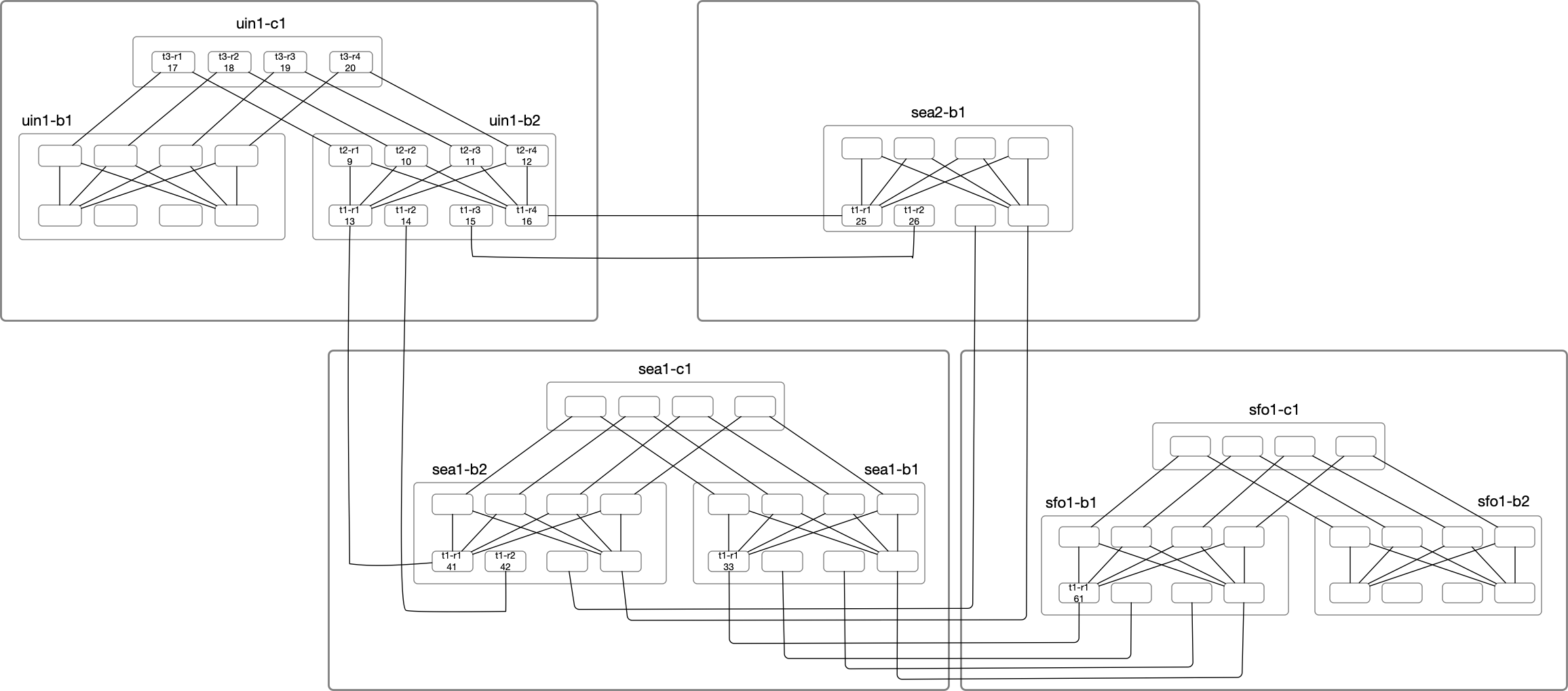In the above diagram, Nodes under uin1-b2 will be the primary focus of our deep dive. Node Label consists of the node name suffix and the last octet of the loopback IP. For example:

uin1-b2-t1-r1 with LSP ID of 0000.0000.0013 is highlighted as t1-r1(13) under uin1-b2 block.
uin1-b2-t2-r1 with LSP ID of 0000.0000.0009 is highlighted as t2-r1(09) under uin1-b2 block.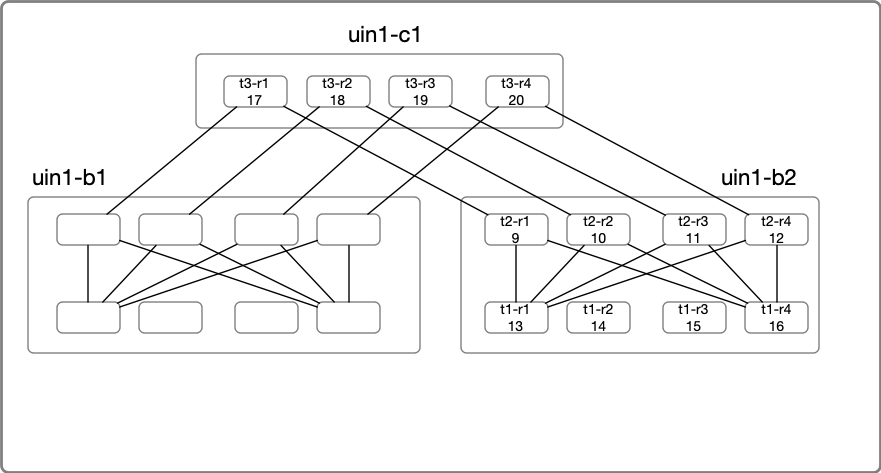## IS-IS Refresher

Let’s do a quick IS-IS refresher. We know that IS-IS Packets are of following types:

## Introduction

In optimization theory, Maximum Flow problems involve finding the maximum flow (or traffic) that can be sent from one place to another, subject to certain constraints. In this post, we will look at Maximum Flow algorithms applied to Networking and the questions they can help answer.

The main focus here will be the applied part, and we will only cover the surface of most algorithms as many of them requires Linear Programming and Optimization theory background.

### Problem Setup

Assume that we have a small network connecting a few locations in the US using RSVP-TE for traffic management.
RSVP-TE allows us to find paths if there is not enough room on the shortest path, which removes the restriction that the flows need to travel only on the shortest path.

In the below picture, we can see the Capacity and IGP cost of the links. From a graph representation perspective, we will use MultiDigraph. Multi to represent multiple links, like between lax<-->iad, and Digraph for capturing the unidirectional behavior of RSVP LSPs.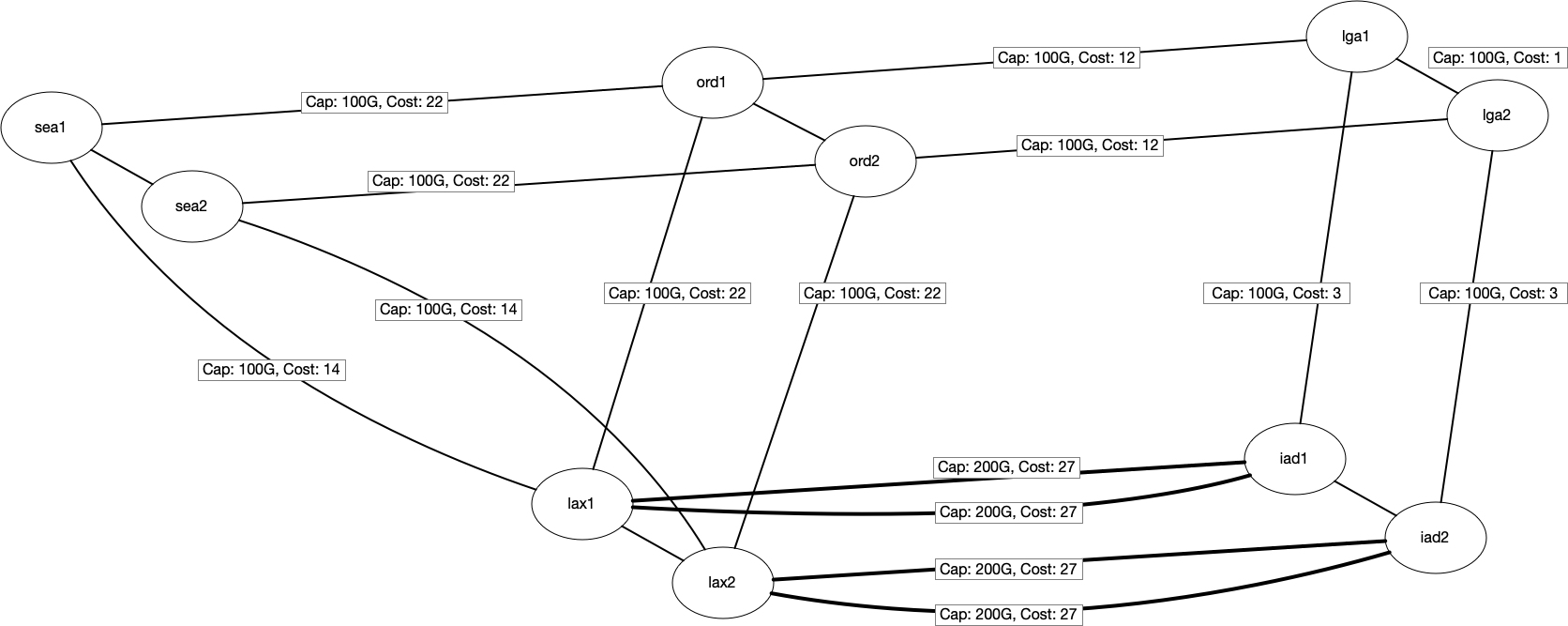We will also assume that we already have some traffic routed between a few locations. The below table shows the existing traffic traveling between locations. For example, we

## Introduction

In the last blog, we looked at SIS/SIR epidemic modeling using Discrete event simulation. This post will cover some fundamental concepts of Discrete Event Simulation, look at a few basic examples to develop an understanding, and end with a simulation of M/M/1 queuing model.

To get started, let’s look at an elementary example.Assume that we want to estimate the probability of observing the head in an experiment of tossing a coin. We know that if the coin is not biased, then the likelihood of getting a head is 1/2. We also know that if I toss the coin two or three times, we may not get exactly 1/2. We expect that if we keep tossing the coin for a very long time, the average probability of getting heads will converge to 1/2.

#### Experiment

So let’s run the experiment 1000 times of tossing the coin, and we get 0.49 as the probability of getting head, which is very close to our expectation of 1/2.

import random
import numpy as np
n = 1000
observed = []

for i in range(n):
observed. 

## Introduction

One of the things I have been trying to play recently with is Discrete Event Simulation(DES). I think it is a powerful tool for validating ideas. In this post, we will look at a toy epidemic model to simulate SIS/SIR models.

In Epidemic modeling, there are two classic models - SIS and SIR models. The models divide the population into different categories corresponding to different stages of the epidemic.

1. Susceptible(S): Susceptible individuals can contract the disease.
2. Infected(I): Infected individuals have already been contracted the disease.
3. Recovered(R): Recovered individuals are recovered from the disease and can not be infected again.

### SIS Model

In case of SIS, the main assumption is that an infected person can get infected again after recovering. The state transition diagram looks like:• $\beta$ is the probability of transitioning from Susceptible(S) to Infected(I)
• $\mu$ is the probability of transitioning from Infected(I) to Susceptible(S)

### SIR Model

In case of SIR, the main assumption is that an infected person can not get infected again. The state transition diagram looks like: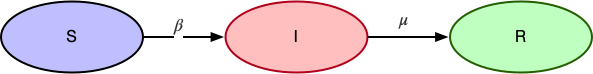• $\beta$ is the probability of transitioning from Susceptible(S) to Infected(I)
• $\mu$ is the probability of transitioning from Infected(I) to Recovered(R)

## SIS Simulation

We will have a generic Simulation class

## Introduction

A system is robust if the failure of some components doesn’t affect its function. As network engineers, we face various types of network failures like link, node failures all the time.

Generally, we use various Network modeling tools like Cariden(WAE), WANDL, etc. to model failures and see how the network reacts under a given failure condition. The components which are in play are:

1. Type and Number of failures. Type: Link, Node. Number of Failures: Single or Double.
2. Routing protocols running on top of the network and there reaction to the failure. Example: RSVP-TE, Pure IGP, SR-TE etc.
3. Network flows and their volume.
4. Network Topology.

In this blog post, we will focus purely on #4 Network topology and certain characteristics of topology, which may make them more robust than other topologies.

## Key Idea

The network topology may have some critical nodes. If we can identify them and take them out of the service, they will significantly impact the functionality of the network. For example, in the case of a Hub and Spoke topology, if a Hub is out of service, it affects all the spokes vs. a spoke out of service. We can make this hub and spoke topology more

## Introduction

You may or may not have already heard about the Five Number summary for a dataset. It’s basically a set of descriptive statistics for a given dataset, which provides an idea about the dataset. Those are:

1. Minimum
2. First quartile
3. Median
4. Third quartile
5. Maximum

Similarly, there are specific statistics about topology, which gives an idea about any network topology. The ones which I think the most essential are:

1. Density and Sparsity
2. Average Degree
3. Assortativity
4. Average Path Length
5. Clustering Coefficient

## Sample Topology

We will be using Cogent topology, which is publicly available here to follow along with our examples. The map represents the nodes in US + Mexico, and European countries.Each node color represents a specific country.Graphml version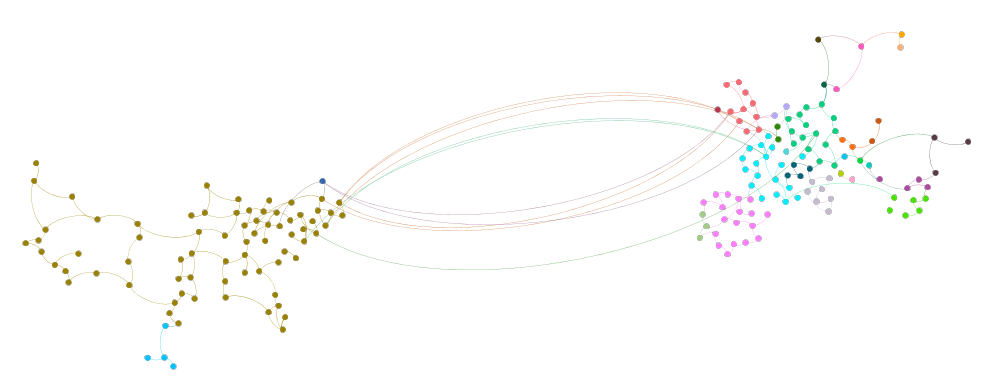You may have already noticed that in the graph, each city is represented as a Node. In reality, any city will have many routers, which will make the topology a lot bigger and more attractive. For our purposes, the current topology abstraction provides the right balance where it’s not huge to overwhelm the reader but big enough to keep things interesting.

## Five Number Summary

### Density and Sparsity

A Graph consists of nodes and links connecting those nodes. An obvious thing to

## Motivation

Clustering is a way to make sense of the data by grouping similar values into a group. There are many ways to achieve that and in this post we will be looking at one of the way based on spectral method. Spectral clustering provides a starting point to understand graphs with many nodes by clustering them into 2 or more clusters. This clustering technique can also be applied for analyzing general data. This technique is based on Linear algebra and Graph theory.

We will start with a very brief introduction of the prerequisite for the sake of completeness and one can skip the prerequisite topics if they already have the familiarity.

## Prerequisite Topic

### Eigen Vectors and Eigen Values

One way to interpret when we multiply a vector a matrix is that a matrix transforms the vector. For example: below is a vector $$\begin{pmatrix} 2\\1 \end{pmatrix}$$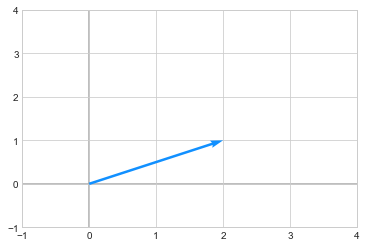we apply a transformation by multiplying the above vector to a matrix

$\begin{pmatrix} -1 & 3 \\ 2 & -2 \end{pmatrix}$

The resultant vector $$\begin{pmatrix} 1\\2 \end{pmatrix}$$ is in orange after transformation.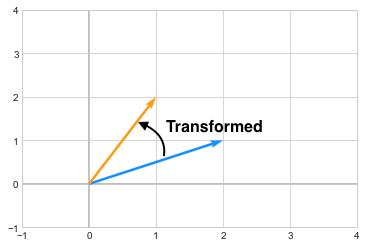you can see how the vector changed its direction after the transformation. Now in case of Eigenvectors, which are special kinds of

## Motivation

Following up from our previous post on Bayesian Finite Mixture Models, here are my notes on Non-Finite mixture model.

## Non-finite Mixture Models

Bayesian finite mixture models can be used when we have a prior knowledge or some good guess on the number of groups present in the dataset. But if we do not know this beforehand, then we can use Non-Finite mixture models. Bayesian solution for this kind of problems is related to Dirichlet process.

## Dirichlet Process(DP)

We briefly mentioned about Dirichlet distribution in the previous post Bayesian Finite Mixture Models, which is a generalization of beta distribution, similarly Dirichlet Process is an infinite-dimensional generalization of Dirichlet distribution. The Dirichlet distribution is a probability distribution on the space of probabilities, while Dirichlet Process is a probability distribution on the space of distributions. A Dirichlet Process is a distribution over distributions. When I first read this, my mind went.

What this means is, that a single draw from a Dirichlet distribution will give us a probability and a single draw from a Dirichlet Process will give us a Dirichlet distribution. For finite mixture models, we used Dirichlet distribution to assign a prior for the fixed number of clusters,

## Motivation

I have been lately looking at Bayesian Modelling which allows me to approach modelling problems from another perspective, especially when it comes to building Hierarchical Models. I think it will also be useful to approach a problem both via Frequentist and Bayesian to see how the models perform. Notes are from Bayesian Analysis with Python which I highly recommend as a starting book for learning applied Bayesian.

## Mixture Models

In statistics, mixture modelling is a common approach for model building. A Model built by simpler distributions to obtain a more complex model. For instance,

• Combination of two Gaussian’s to describe a bimodal distribution.
• Many Guassian’s to describe any arbitrary distributions.

We can use a mixture of models for modelling sub-populations or complicated distributions which can not be modelled with simpler distributions.

## Finite Mixture Models

In Finite mixture models, as the name suggests, we mix a known number of models together with some weights associated for each model. Probability density of the observed data is a weighted sum of the probability density for K subgroups of the data where K is the number of models.

$p(y|\theta) = \sum_{i=1}^{K} w_{i}p_{i}(y_{i}|\theta_{i})$

Here, $$w_{i}$$ is the weight for each group and all the

## Motivation

I recently observed a conversation around ECMP/Hash buckets which made me realize on how the end to end concept is not very well understood. So this provided me enough motivation to write about this topic which will be covered in various upcoming blog posts. But while thinking about the subject, I ran into an interesting RFC RFC2992. This RFC goes through a simple mathematical proof which I found impressive due to the fact that someone wrote that in ASCII in 2000. My intent in this blog post is to provide some colorization to the RFC and perhaps cover a bit more in detail.

## Introduction

In the RFC, the focus is on Hash-threshold implementation for mapping hash values to the next-hop. To re-iterate for completeness sake, we all know that a router computes a hash key based on certain fields, like SRC IP, DST IP, SRC Port, DST Port by performing a hash (CRC16, CRC32, XOR16, XOR32 etc.). This hash gets mapped to a region and the next-hop assigned to that region is where the flow get’s assigned.

For example,assume that we have 5-next hops to choose from and we have a key space which is 40 bits wide.